A LEVEL-SET APPROACH FOR THE
METAMORPHOSIS OF SOLID MODELS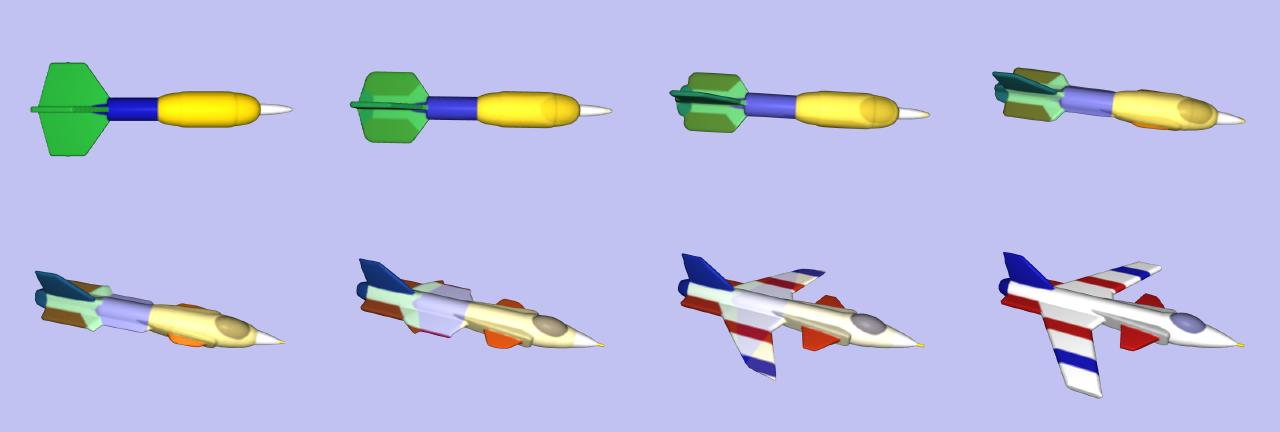David E. Breen Computer Graphics Laboratory MS 348-74 California Institute of Technology Pasadena, CA 91125 Tel (626) 395-2866 FAX (626) 793-9544 david@gg.caltech.edu Ross T. Whitaker School of Computing 4540 Merrill Engineering Building University of Utah Salt Lake City, UT 84112 Tel (801) 587-9549 FAX (801) 585-6513 whitaker@cs.utah.edu

INTRODUCTION

One form of metamorphosis (morphing) is 3-D morphing (or shape morphing), which involves smoothly changing a 3-D source object (model) into a target object. We present a new approach to this task, that employs an active deformable surface which starts at the source shape and smoothly changes into the target shape. (See Breen & Whitaker 2001, and Whitaker & Breen 1998). This deformation process is described by the optimization of an objective function that measures the similarity between the target and the deforming surface. We represent the deformable surface as a level set (iso-surface) of a densely sampled scalar function of 3 dimensions. The sampling produces a regularly-spaced rectilinear volume dataset. Such level set models have been shown to mimic conventional parametric deformable surface models by encoding surface movements as changes in the grey-scale values of the volume. A well-founded mathematical structure leads to a set of procedures that describes how voxel values can be manipulated to create deformations in the level sets. The result is a voxel-based modeling technology that offers several advantages over previous methods, including support for a wide range of user input, no need for parameterization, flexible topology, and sub-voxel accuracy.

This work is based on the image morphing strategy, which consists of two distinct steps: global geometric warping and blending. The former step utilizes a coordinate transformation that maps the source model into approximately the same shape and orientation as the target model. The coordinate transformation can take a variety of different forms, among the most successful of which has been shown in the literature to be a combination of a rigid transformation and a non-rigid, spline-based coordinate map. However, our major concern is the second step, blending. A blending completes the morph by ensuring that any discrepancies that remain in the images/volumes after the warping are gradually removed over the course of the morphing process. Our work focuses primarily on developing a superior method for the blending stage, one which reduces the amount of user input required to produce an acceptable morphing result. Indeed, the proposed level set approach to shape metamorphosis will produce a reasonable morph with virtually no user input. Given this technology, user input can be incorporated in an incremental fashion starting with a minimal amount and proceeding with progressively more until a satisfactory result is achieved.

Our level set approach to 3-D model morphing provides several advantages over other 3-D morphing algorithms. Level set models are active, since their underlying motion is defined algorithmically rather than interactively. The amount of user input may range from virtually none to a full 3-D warping description of the process, and the results are reasonable with any intermediate amount of user input. Level sets do not utilize parameterization, so our method will not lead to any limitations that usually come with parameterization, e.g. a limited set of possible shapes and the need for reparameterization after undergoing significant changes in shape. This lack of parameterization allows for a change of topology of the object while morphing. For example, the object may split into pieces to form multiple objects, or vice versa. Finally, both the scan conversion and deformation stages of our morphing approach produce models with user-defined sub-voxel accuracy.

METAMORPHOSIS AS A GOAL-DRIVEN PROCESS

The proposed strategy for object metamorphosis is based on the following principle: Shape metamorphosis is the process by which one object seeks to resemble the other. This philosophy raises two questions. First, what is the metric by which we can quantify the similarity of two objects? Second, what is the process by which one object seeks to optimize that metric? It turns out that even very simple answers to these questions produce very powerful algorithms for shape metamorphosis, with a great deal of opportunity for future enhancement and refinement of the resulting algorithms.

In order to create a very general algorithm for metamorphosis, we work with a very general notion of a surface. Consider an open set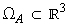, which is the source object, and a target,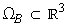. The source is enclosed by a surface SA =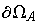, and likewise SB =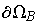. We propose a very simple metric for comparing two shapes that maximizes the volume shared by the interiors of the two objects. First, define an inside-outside function for the target,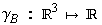, such that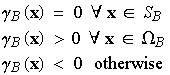.

In words, the inside-outside function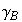is zero on the surface of the object, positive on the inside, and negative on the outside. This function can be used to quantify the extend to which an indeterminate object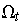overlaps with the target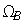with the volume integral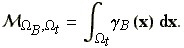Notice that this integral achieves its maximum,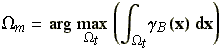,

when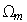=, since in that case the integral includes all of the positive parts ofand none of the negative parts. We can compute the first variation of this metric with respect toby noting that incremental changes in the object shape can be expressed in terms of the surface that encloses it: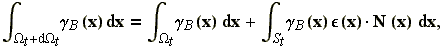where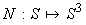is the surface normal, which is a mapping from every point on the surface to the unit sphere. Differentiating with respect to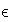gives the first variation with respect to surface position: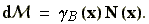This result confirms that local maxima exist only where the surface S lies on the zeros of. Thus, for a local maximum with respect towe can conclude that each connected component ofis either exactly matched by a corresponding piece ofor it is missing, i.e. there is no corresponding component to. This formulation suggests a strategy for shape metamorphosis: Construct a family of objects(with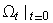=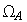) that evolve according to a hill-climbing strategy, and maximize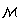. If initialized in such a way that all of the  connected components ofhave some overlap with, then the deformation will gradually seek the global maximum, which is the target.

The intermediate shapes independ, of course, on the choice of the function. In order to avoid numerical difficulties, it should be continuous. Furthermore,should reward shapes that are similar to the target but offset by some small distance. A natural choice ofis a signed distance transform of the target surface SB or some monotonic function thereof. Thus, if we let a function DB be the signed distance transform, then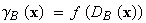, where f(0) = 0, and f'(a) > 0. By tuning f, one can control the way the metamorphosis behaves when intermediate surfaces are far from the target. If f(a) = a, then St contracts or expands with a magnitude that depends on the signed distance to the target.

As discussed earlier, this low-level process is meant to address the blending stage of 3-D morphing. It can be combined with some higher-level process that accounts for "semantic" aspects of shape correspondence. Only users can supply such semantic considerations. In the event that the user is not able or willing to define every important correspondence between two objects, some other method must "fill in" the gaps remaining between the source and target surface. To this end, we propose the use of free-form deformations, implemented with level set models, to achieve a continuous transition between shapes that result from the underlying coordinate transformation.

LEVEL SET MODELS

In practice, the strategy of shape metamorphosis by surface deformations described above must be computed using some specific surface representation. Ideally, we would like the surface representation to be as general as the underlying theory. For this we use the method of level set models, which is a technique for modeling surfaces as isovalues of a densely sampled scalar function of the domain. Surface movements are encoded as changes in the grey-scale values of the voxels. Using level set models, we can compute goal-driven deformations on surfaces without any explicit surface representation.

Level set models represent a set of surface points S as an isosurface of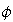, which we will call the embedding.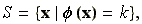where the value of k is arbitrary and will fall out of subsequent calculations (subsequently, we will set k = 0). We can also represent a family of surfaces and a corresponding family of embeddings: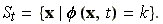Consider a point S(t) on the surface that moves through space as a function of t. Because S(t) remains the kth level-surface ofover time, the derivative ofwith respect to time must be zero. Thus,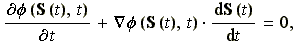which establishes the connection between the way points on St move and the way the greyscale values (at positions on the surface) of the embedding change: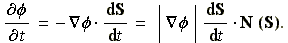Using the first variation established in the preceding section and a hill climbing strategy, we have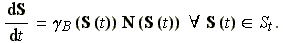For the embedding, this gives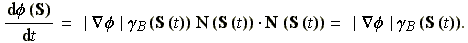Notice, we have not chosen a particular k, and therefore this analysis applies to every level set of, and thus we are describing the deformation of an embedded family of surface models, each of which evolves according to the same equation: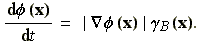The particular level set of interest is the one that we choose, by construction of the initial conditions, such that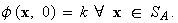The complete deformation strategy is as follows. First, initialize a volume so that the kth level set is, approximately, aligned with SB. For all our work, we will use the zero-set as the level set model, using the discrete distance transform of SB. We use this initialization to solve the initial value problem given by the preceding equation, using the distance transform of the target as the, which forces the level set model toward the goal. We solve this equation using finite forward differences. When the model is sufficiently close to the target (a threshold on the RMS distance to the target), the process stops and the metamorphosis is complete.

3-D METAMORPHOSIS

The specific steps of our level set morphing approach are (1) 3-D scan conversion of source and target objects, (2) application of coordinate transformations, (3) level set deformation, and (4) polygonization and rendering.

The first step, 3-D scan conversion, is detailed at the following web page:
http://www.gg.caltech.edu/~david/3D_scan_conv.html
This step produces the two volume datasets needed for the next stages. The first volume represents the source object and provides the initial conditions for the deformation stage. The second volume is a sampling of the signed distance transform of the target object, which is used to drive the deformation process.

In order for our active level set model to deform from one surface into another the source and target objects must overlap. The objects may be automatically or interactively positioned, as well as, interactively warped in order to produce a particular model alignment. The user may choose any of these methods depending upon the level of control and final output desired. The source object will shrink in those areas where it is outside the target object, and will expand in those areas inside the target model. Thus, the user controls the morph by defining the regions of overlap between the source and the target. This is accomplished by applying a coordinate transformation which maps the voxel locations of the source object into new locations in the target object's distance volume. The transformation is given by

x' = T (x,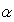),

where 0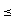1 parameterizes a continuous family of transformations that begins with identity, i.e. x = T (x, 0), and smoothly becomes the user-defined transformation at T (x, 1). The parameterization is utilized during polygonization and discussed later. For this work, we have developed a software tool that allows a user to interactively position, rotate and scale the source and target objects in order to produce the transformation T. A generalized warping, with extensive user input, may also be utilized to provide an even more detailed control of the process.

Step (3) (level set deformation) was described in the previous section.

Finally, the viewing of the morph can be achieved by extracting a polygonal iso-surface (using the Marching Cubes algorithm) from each volume produced by the level set deformation process. The polygons are rendered to produce a series of images, which are then combined to produce an animation. The algorithm used to color shade our level set models has been described in Breen, Mauch & Whitaker 1999, 2000.

RESULTS

Still images and animations from our morphing sequences may be found at the following URLs: http://www.gg.caltech.edu/~david/morph_results.html.
http://www.gg.caltech.edu/~david/eg_morph.html.